﻿ 高速公路收费站通行能力研究 Research on Expressway Toll Capacity

Vol.07 No.04(2018), Article ID:24616,7 pages
10.12677/AAM.2018.74045

Research on Expressway Toll Capacity

Wushuang Tan1*, Yi Yuan1, Yi Lei2

1College of Mathematics and Software Science, Sichuan Normal University, Chengdu Sichuan

2College of Computer Science, Sichuan Normal University, Chengdu Sichuan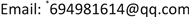Received: Apr. 8th, 2018; accepted: Apr. 20th, 2018; published: Apr. 27th, 2018ABSTRACT

In this paper, the duplicate toll plazas and fan toll plazas are investigated through the analysis of express toll access and fan toll access, respectively. The mathematical models are found to discuss the optimum construction scheme under different traffic flows and the toll plaza area of different shapes. And then the result is obtained that the area of duplicate toll plaza is smaller than that of the fan toll plaza. Finally, it is found that the conversion of the traditional man-made toll form into MTC and ETC substantially improves the throughput of the toll station.

Keywords:Duplicate Toll Access, Fan-Shaped Toll Access, Toll Plaza

1四川师范大学数学与软件科学学院，四川 成都

2四川师范大学计算机科学学院，四川 成都1. 引言

2. 复式收费通道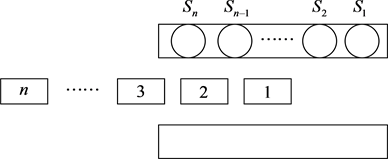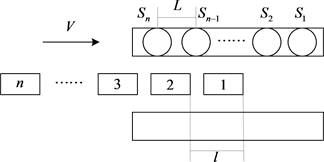${U}_{i}={y}_{i}+{Q}_{i}=i×t+\frac{\left(m-i\right)×L+i×l}{v}$

${W}_{k}=\mathrm{max}\left({T}_{1}\cdots {T}_{n}\right)+R=\left(m-k\right)×t+\frac{\left(m-k\right)×L+k×l}{v}+k×t+{S}_{k}.$

3. 扇形式收费通道$\left\{\begin{array}{l}{p}_{n}=\frac{{\left({\rho }^{\prime }\right)}^{n}}{n!}{p}_{0},\text{\hspace{0.17em}}\text{\hspace{0.17em}}\text{\hspace{0.17em}}\text{\hspace{0.17em}}\text{\hspace{0.17em}}0\le n\le C,\\ {p}_{n}=\frac{{\left({\rho }^{\prime }\right)}^{n}}{{C}^{n-C}n!}{p}_{0},\text{\hspace{0.17em}}\text{\hspace{0.17em}}\text{\hspace{0.17em}}n\ge C.\end{array}$

$E\left(T\right)=E\left(W\right)+E\left(S\right)$

4. 成本分析

$Y={\beta }_{0}+{\beta }_{1}×{x}_{1}+{\beta }_{2}×{x}_{2}+{\beta }_{3}×{x}_{3}$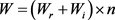，其中n为收费站个数，主路宽度d，梯形长度 $L=\frac{W-d}{2\mathrm{tan}\alpha }$ ，收费岛长度l。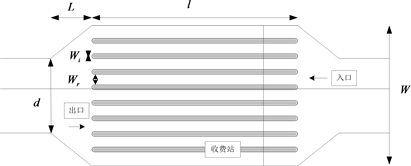4.1. 复式收费广场

$S=\frac{\left(W+d\right)L}{2}+W\left(l+{l}^{\prime }\right)$

4.2. 扇形收费广场

$S=\frac{\left(W+d\right)L}{2}+Wl$

5. 模型求解

X为收费站设计和交通运行参数矩阵， ${Y}_{i}\left(i=1,2,3,4\right)$ 代表第i个样本的收费站年交通事故次数，β为系数矩阵，则样本可用下面的矩阵表示：

$X=\left[\begin{array}{cccc}1& {x}_{1,1}& {x}_{1,2}& {x}_{1,3}\\ 1& {x}_{2,1}& {x}_{2,2}& {x}_{2,3}\\ 1& {x}_{3,1}& {x}_{3,2}& {x}_{3,3}\\ 1& {x}_{4,1}& {x}_{4,2}& {x}_{4,3}\end{array}\right],\text{\hspace{0.17em}}Y=\left[\begin{array}{c}{Y}_{1}\\ {Y}_{2}\\ {Y}_{3}\\ {Y}_{4}\end{array}\right],\text{\hspace{0.17em}}\beta =\left[\begin{array}{c}{\beta }_{0}\\ {\beta }_{1}\\ {\beta }_{2}\\ {\beta }_{3}\end{array}\right]$

5.1. 复式收费广场

$\stackrel{^}{Y}={\stackrel{^}{\beta }}_{0}+{\stackrel{^}{\beta }}_{1}×{x}_{1}+{\stackrel{^}{\beta }}_{2}×{x}_{2}+{\stackrel{^}{\beta }}_{3}×{x}_{3}$

$\left(\begin{array}{c}{x}_{11}\\ {x}_{21}\\ {x}_{31}\\ {x}_{41}\end{array}\right)=\left(\begin{array}{c}\text{50}\\ \text{3}3\\ \text{25}\\ \text{20}\end{array}\right)$$\left(\begin{array}{c}{x}_{12}\\ {x}_{22}\\ {x}_{32}\\ {x}_{42}\end{array}\right)=\left(\begin{array}{c}0.70\\ 0.77\\ 0.84\\ 0.90\end{array}\right)$$\left(\begin{array}{c}{x}_{13}\\ {x}_{23}\\ {x}_{33}\\ {x}_{43}\end{array}\right)=\left(\begin{array}{c}0.010\\ \begin{array}{l}0.013\\ 0.016\end{array}\\ 0.020\end{array}\right)$ 。对系数矩阵标准化处理后进行极大似然估计，可得到一个正规方程组： ${X}^{\text{T}}X\beta ={X}^{\text{T}}Y$ 。β的极大似然估计为：

$\stackrel{^}{\beta }={\left({X}^{\text{T}}X\right)}^{-1}{X}^{\text{T}}Y=\left(\begin{array}{c}1.1832\\ 7.3015\\ -4.8059\\ \text{3}\text{.3212}\end{array}\right)$

$\stackrel{^}{Y}={\stackrel{^}{\beta }}_{0}+{\stackrel{^}{\beta }}_{1}×{x}_{1}+{\stackrel{^}{\beta }}_{2}×{x}_{2}+{\stackrel{^}{\beta }}_{3}×{x}_{3}=1.1832+7.3015×{x}_{1}-4.8059×{x}_{2}+3.3212×{x}_{3}$

${X}^{\prime }={\left(\begin{array}{ccc}20.000& 8.00& 0.015\end{array}\right)}^{\text{T}}$ 标准化得到的数据带入回归方程得到 ${\stackrel{⌢}{Y}}^{\prime }=13$

5.2. 扇形收费广场

$\left(\begin{array}{c}{x}_{11}\\ {x}_{21}\\ {x}_{31}\\ {x}_{41}\end{array}\right)=\left(\begin{array}{c}\text{20}\\ \text{17}\\ \text{14}\\ \text{13}\end{array}\right)$$\left(\begin{array}{c}{x}_{12}\\ {x}_{22}\\ {x}_{32}\\ {x}_{42}\end{array}\right)=\left(\begin{array}{c}0.70\\ 0.77\\ 0.84\\ 0.90\end{array}\right)$$\left(\begin{array}{c}{x}_{13}\\ {x}_{23}\\ {x}_{33}\\ {x}_{43}\end{array}\right)=\left(\begin{array}{c}0.010\\ \begin{array}{l}\text{0}.013\\ 0.016\end{array}\\ 0.020\end{array}\right)$ 。对系数矩阵进行极大似然估计，可得到一个正规方程组： ${X}^{\text{T}}X\beta ={X}^{\text{T}}Y$ 。则β的极大似然估计为：

$\stackrel{^}{\beta }={\left({X}^{\text{T}}X\right)}^{-1}{X}^{\text{T}}Y={\left(\begin{array}{cccc}-3.0023& 1.5195& 3.7419& -2.2581\end{array}\right)}^{\prime }$

$\stackrel{^}{Y}={\stackrel{^}{\beta }}_{0}+{\stackrel{^}{\beta }}_{1}×{x}_{1}+{\stackrel{^}{\beta }}_{2}×{x}_{2}+{\stackrel{^}{\beta }}_{3}×{x}_{3}=-3.0023+1.5195×{x}_{1}+3.7419×{x}_{2}-2.2581×{x}_{3}$

${X}^{″}={\left(\begin{array}{ccc}20.000& 8.00& 0.015\end{array}\right)}^{\text{T}}$ 标准化后带入回归方程得到 ${\stackrel{⌢}{Y}}^{″}=30$

5.3. 成本分析模型求解

 在本文的假设中，当在设计收费广场时，若更看重道路的吞吐量，综合考虑，使用扇形收费站更佳。

 根据实际情况给吞吐量，事故预防和土地成本赋予不同的权重，则得到相适应的解决方案。

6. 仿真方案设定

 当ETC使用率为30%时，可知：当交通流量 < 1000 veh/h时，配置1条ETC通道收费服务水平最佳，即ETC和MTC的比率为1:3；当交通流量 > 1000 veh/h时，配置2条ETC通道服务水平最佳，即ETC和MTC的比率为1:1。

 当ETC使用率为60%时，可知：当交通流量 < 1400 veh/h时，配置1条ETC通道收费服务水平最佳，即ETC和MTC的比率为1:3；当交通流量 > 1400 veh/h时，配置2条ETC通道收费服务水平最佳，即ETC和MTC的比率为1:1。

 当ETC使用率为90%时，可知：配置3条ETC通道收费站服务水平最佳，配置2条也可，即ETC和MTC的比率为3:0或2:1。

Research on Expressway Toll Capacity[J]. 应用数学进展, 2018, 07(04): 362-368. https://doi.org/10.12677/AAM.2018.74045

1. 1. 程俊龙. ETC-MTC混合式收费站通行能力研究[D]: [硕士学位论文]. 成都: 西南交通大学, 2015.

2. 2. 田根平. 高等级公路收费站通行能力评价研究[D]: [硕士学位论文]. 昆明: 昆明理工大学, 2008.

3. 3. 刘伟铭. 道路收费系统的优化方法研究[D]: 博士学位论文. 北京: 国防科学技术大学, 2004.

4. 4. Kai, N. and Schreckenberg, M. (1992) A Cellular Automaton Model for Freeway Traffic. Journal De Physique I, 2, 2221-2229.

5. 5. 张晨琛, 王艳辉, 贾利民. ETC系统对收费站服务水平的影响研究[J]. 交通运输系统工程与信息, 2013, 13(2): 147-152.

6. NOTES

*通讯作者。